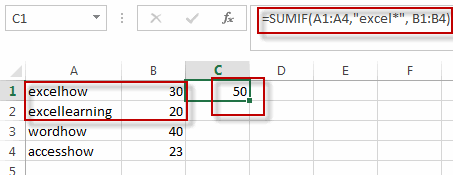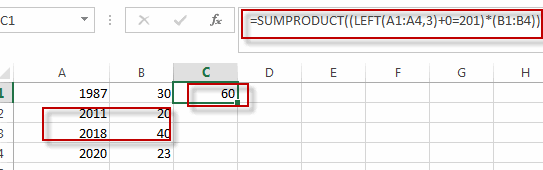# Sum if Cells Begin with Specific Text or Number in Another Cells

This post will guide you how to sum cells if cells begin with a specific text or number in another column in excel. How do I sum if cells start with specific text or number in another cells in excel.

Assuming that you have a list of data A1:B4, and you want to sum all cells in column B if cells in column A begin with a specific text “excel” or a specific number “201”, how to achieve it.

## Sum if Cells Begin with Specific Text or Number

If you want to sum cells in column B when the adjacent cells in column A start with a specific test “excel”, you can create a formula based on the SUMIF function. like this:

`=SUMIF(A1:A4,"excel*", B1:B4)`This function will sum cells in range B1:B4, but it must be match the given criteria that cells in A1:A4 start with the specific text “excel”.

If you want to sum cell in column b when the adjacent cell in column A start with a specific number “201”, and you also can create a formula based on the SUMPRODUCT function and the LEFT function. Like this:

`=SUMPRODUCT((LEFT(A1:A4,3)+0=201)*(B1:B4))`The LEFT function will extract the first 3 numbers, cos the number 201 have three digits.

### Related Functions

• Excel SUMPRODUCT function
The Excel SUMPRODUCT function multiplies corresponding components in the given one or more arrays or ranges, and returns the sum of those products.The syntax of the SUMPRODUCT function is as below:= SUMPRODUCT (array1,[array2],…)…
• Excel SUMIF Function
The Excel SUMIF function sum the numbers in the range of cells that meet a single criteria that you specify. The syntax of the SUMIF function is as below:=SUMIF (range, criteria, [sum_range])…
• Excel LEFT function
The Excel LEFT function returns a substring (a specified number of the characters) from a text string, starting from the leftmost character.The LEFT function is a build-in function in Microsoft Excel and it is categorized as a Text Function.The syntax of the LEFT function is as below:= LEFT(text,[num_chars])…t)…